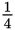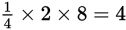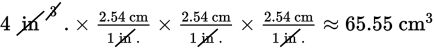# SAT Math Multiple Choice Question 834: Answer and Explanation

### Test Information

Question: 834

MetalDensity (g/cm3)
Copper8.96
Iron7.87
Nickel8.90
Tin7.26

9. The density of a substance can be found by dividing the mass of the substance by the volume ofthe substance. The table gives the density of several pure metals in grams per cubiccentimeter. Suppose a rectangular sheet of a pure metal weighs about 515.9 grams andmeasuresinch by 2 inches by 8 inches. Assuming the sheet is one of the metals in the table,which metal is it? (There are approximately 2.54 centimeters in 1 inch.)

• A. Copper
• B. Iron
• C. Nickel
• D. Tin

Explanation:

B

Difficulty: Medium

Category: Problem Solving and Data Analysis / Rates, Ratios, Proportions, and Percentages

Strategic Advice: Always pay careful attention to units. When converting from a linear measure, such as inches, to a cubic measure, such as cm3 (or vice versa), you will need to write three unit conversions.

Getting to the Answer: Start by finding the volume of the metal sheet using the formula V = lwh. The volume iscubic inches. Now, carefully convert this to cubic centimeters:Skim the question again: To find the density of the metal, divide the mass (given in the question) by the volume: 515.9 ÷ 65.55 ≈ 7.87, which matches the density of iron, (B).